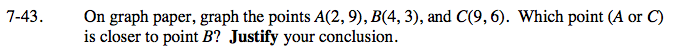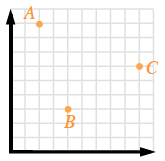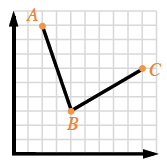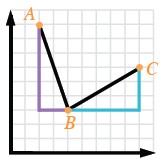### Home > GC > Chapter 7 > Lesson 7.1.4 > Problem7-43

7-43.

On graph paper, graph the points A(2, 9), B(4, 3), and C(9, 6). Which point (A or C) is closer to point B? Justify your conclusion. Homework Help ✎Plot the points.

Draw lines to show the distance between points A and B and between points C and B.

Draw slope triangles for both line segments.

Use the Pythagorean Theorem to find the lengths of the line segments.